# NCERT Solutions for Class 10 Maths Chapter 9 Some Applications of Trigonometry

NCERT solutions for class 10 maths chapter 9 Some Applications of Trigonometry- This is an application chapter of the previous chapter. In this particular chapter, we will study some ways in which trigonometry is used in our daily life. Solutions of NCERT class 10 maths chapter 9 Some Applications of Trigonometry has covered the detailed explanation to each and every question. In the previous class, you have studied trigonometric ratios. Trigonometry is used in navigation, geography, construction of maps, determining the position of an island, etc. In this chapter, we will learn how trigonometry is used for finding the distances and heights and various objects, without actually measuring them. Trigonometry is one of the most ancient topics studied by scholars all over the world. In this chapter, there is only one exercise with 16 questions, based on the practical application of trigonometry. CBSE NCERT solutions for class 10 maths chapter 9 Some Applications of Trigonometry are designed by the subject experts to help students in their preparation of board exams. This chapter introduces new terms like the line of sight, angle of depression and angle of elevation. NCERT solutions for class 10 maths chapter 9 Some Applications of Trigonometry will give you assistance while practicing the questions of the practice exercises including optional exercise. Let's understand a few terms used in this chapter through the diagram.

• The line of sight- It is an imaginary line drawn from the observer's eye to the object viewed by the observer.

• The angle of elevation-  The angle of elevation of an object viewed by an observer, is the angle formed by the line of sight with the horizontal level when the object is above the horizontal level,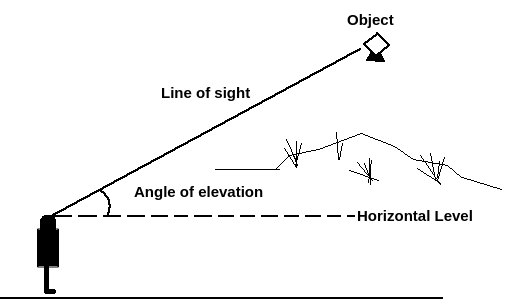• The angle of depression- The angle of depression of an object viewed by an observer, is the angle formed by the line of sight with the horizontal level when the object is below the horizontal level.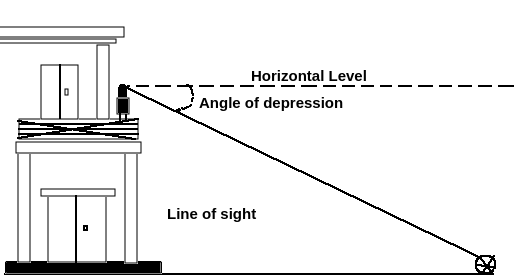Along with this particular chapter, NCERT solutions are available subject wise and chapter wise. If you want to go through them then click on the link given.

## NCERT Solutions for Class 10 Maths Chapter 9 Some Applications of Trigonometry Excercise: 9.1Given that,
The length of the rope (AC) = 20 m. and
Let the height of the pole (AB) be

So, in the right triangle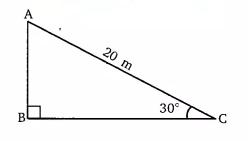By using the Sin rule

m.
Hence the height of the pole is 10 m.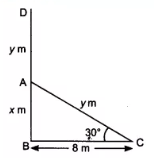Suppose DB is a tree and the AD is the broken height of the tree which touches the ground at C.
GIven that,
, BC = 8 m
let AB =  m and AD =

In right angle triangle ,

So,  the value of  =

Similarily,

the value of  is

So, the total height of the tree is-

= 8 (1.732) = 13.856 m (approx)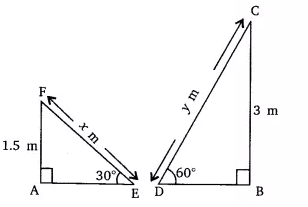Suppose  m is the length of slides for children below 5 years and the length of slides for elders children be  m.

Given that,
AF = 1.5 m, BC = 3 m,  and

In triangle EAF,

The value of  is 3 m.

Similarily in CDB,

the value of  is  = 2(1.732) = 3.468

Hence the length of the slide for children below 5 yrs. is 3 m and for the elder children is 3.468 m.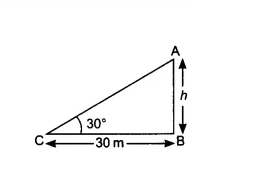Let the height of the tower AB is  and the angle of elevation from the ground at point C is
According to question,
In the right triangle ,

the value of  is  = 10(1.732) = 17.32 m
Thus the height of the tower is 17.32 m

A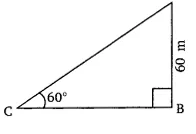Given that,
The length of AB = 60 m and the inclination of the string with the ground at point C is .
Let the length of the string AC be .
According to question,
In right triangle CBA,

The value of length of the string ()  is  = 40(1.732) = 69.28 m
Hence the length of the string is 69.28 m.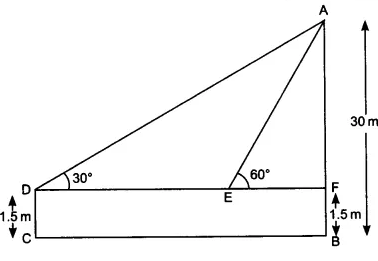Given that,
The height of the tallboy (DC) is 1.5 m and the height of the building (AB) is 30 m.
and

According to question,
In right triangle AFD,

So, DF =

In right angle triangle ,

EF =

So, distance walked by the boy towards the building = DF - EF =Suppose BC =  is the height of transmission tower and the AB be the height of the building and  AD is the distance between the building and the observer point (D).
We have,
AB = 20 m, BC =  m and AD =  m
and

According to question,
In triangle  BDA,

So,  = 20 m

Again,

Answer- the height of the tower is 14.64 mLet the height of the pedestal be  m. and the height of the statue is 1.6 m.
the angle of elevation of the top of the statue and top of the pedestal is(  )and( ) respectively.

Now,
In triangle ,

therefore,  BC =  m

In triangle ,

the value of   is  m
Hence the height of the pedestal is  mIt is given that, the height of the tower (AB) is 50 m.  and
Let the height of the building be  m

According to question,
In triangle PBQ,

.......................(i)

In triangle ABQ,

.........................(ii)
On equating the eq(i) and (ii) we get,

therefore,  = 50/3 = 16.66 m = height of the building.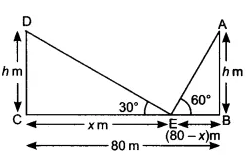Given that,
The height of both poles are equal  DC = AB. The angle of elevation of of the top of the poles are  and  resp.
Let the height of the poles be   m and CE =  and BE = 80 -

According to question,
In triangle DEC,

..............(i)

In triangle AEB,
..................(ii)
On equating eq (i) and eq (ii), we get

m
So,  = 60 m

Hence the height of both poles is ()m and the position of the point is at 60 m from the pole CD and 20 m from the pole AB.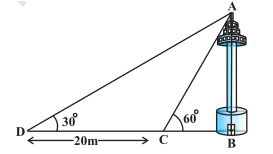Suppose the  is the height of the tower AB and BC =  m
It is given that, the width of CD is 20 m,
According to question,

In triangle ,
............(i)

In triangle ACB,
.............(ii)

On equating eq (i) and (ii) we get:

from here we can calculate the value of    and the width of the canal is 10 m.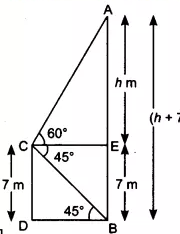Let the height of the cable tower be (AB = )m
Given,
The height of the building is 7 m and angle of elevation of the top of the tower  , angle of depression of its foot .

According to question,

In triangle ,

since DB = CE = 7 m

In triangle ,

Thus, the total height of the tower equal to

Given that,
The height of the lighthouse (AB) is 75 m from the sea level. And the angle of depression of two different ships are  and  respectively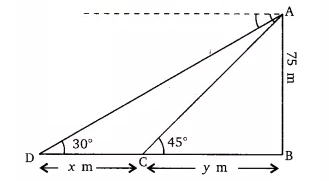Let the distance between both the ships be  m.
According to question,

In triangle ,

.............(i)

In triangle ,

.............(ii)

From equation (i) and (ii) we get;

Hence, the distance between the two ships is approx 55 m.Given that,
The height of the girl is 1.2 m. The height of the balloon from the ground is 88.2 m and the angle of elevation of the balloon from the eye of the girl at any instant is () and after some time .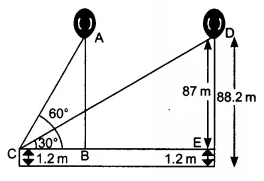Let the  distance travelled by the balloon from position A to position D during the interval.
AB = ED = 88.2 - 1.2 =87 m

Now, In triangle ,

In triangle ,

Thus, distance traveled by the balloon from position A to D

m